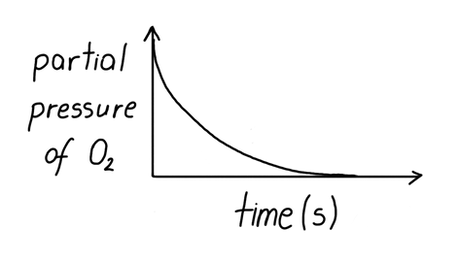# Calculations for SQA Higher Chemistry1. Rate of reaction
2. Rate of reaction
3. Rate of reaction

Amount of product gained or amount of reactant lost can be used to calculate the rate of reaction and draw graphs to represent its change.These graphs can be produced from data sets acquired during practical experiments. Remember when calculating rate of reaction time should be in seconds.The rate of reaction of an experiment is given by the gradient of its graph by finding the change in the mass or the volume of the reactants used or products used per unit time.# 1

What would be an appropriate measurement for the rate of reaction in the reaction between calcium carbonate and hydrochloric acid?

Either loss of mass of the solution or the volume of carbon dioxide produced per unit time could be used to measure the rate of reaction.

mass loss/volume of gas per unit time# 2

How can rate of reaction be calculated by measuring the volume of gas produced by a reactant mixture containing zinc and hydrochloric acid?

Gas produced is hydrogen and may be collected in a gas syringe or under water. Rate of reaction = volume of hydrogen produced / time# 3

Draw a graph to show how the partial pressure of oxygen changes during a reaction 2NO(g) + O₂(g) → N₂O₄(g).

image# 4

Calculate the initial rate of reaction for the graph shown in cm³/s.

0.067 cm³/s# 5

How can rate of reaction be calculated by measuring the mass of a reactant mixture containing zinc and hydrochloric acid?

Mass will decrease as hydrogen is produced and leaves the reactant mixture.
rate of reaction = mass decrease ÷ time

mass decrease ÷ timeEnd of page